# R: Decision Trees (Regression)

Decision Trees are popular supervised machine learning algorithms. You will often find the abbreviation CART when reading up on decision trees. CART stands for Classification and Regression Trees.

In this example we are going to create a Regression Tree. Meaning we are going to attempt to build a model that can predict a numeric value.

We are going to start by taking a look at the data. In this example we are going to be using the Iris data set native to R. This data set

`iris`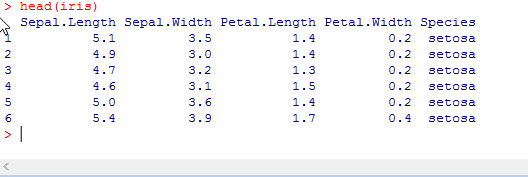As you can see, our data has 5 variables – Sepal.Length, Sepal.Width, Petal.Length, Petal.Width, and Species. The first 4 variables refer to measurements of flower parts and the species identifies which species of iris this flower represents.

In the Classification example, we tried to predict the Species of flower. In this example we are going to try to predict the Sepal.Length

In order to build our decision tree, first we need to install the correct package.

```install.packages("rpart")

library(rpart)```

Next we are going to create our tree. Since we want to predict Sepal.Length – that will be the first element in our fit equation.

```fit <- rpart(Sepal.Length ~ Sepal.Width + Petal.Length + Petal.Width+ Species,
method="anova", data=iris )```

Note the method in this model is anova. This means we are going to try to predict a number value. If we were doing a classifier model, the method would be class.

Now let’s plot out our model

```plot(fit, uniform=TRUE,
main="Regression Tree for Sepal Length")
text(fit, use.n=TRUE, cex = .6)```

Note the splits are marked – like the top split is Petal.Length < 4.25

Also, at the terminating point of each branch, you see and n= . The number following this is the number of elements from the data file that fit at the end of that branch.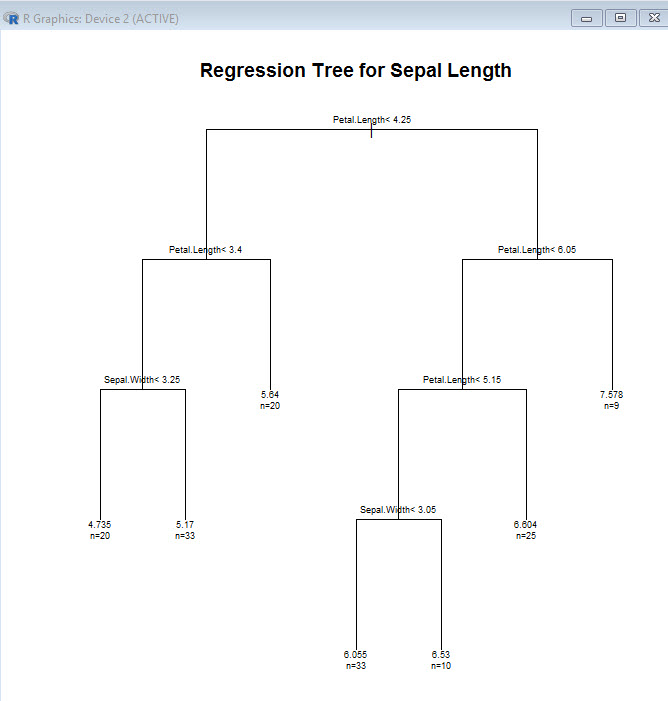While this model actually works out pretty good, one thing to look for is over fitting. A good sign of that would be having a bunch of branches terminating with n values of 1 or 2. This means the model is tuned too much to the test data and when run up against a new set of data it will most likely result in poor predictions.

Of course we can look at some of the numbers if you are so inclined.Notice the xerror (cross validation error) gets better with each split. That is something you want to look out for. If that number starts to creep up as the splits increase, that is a sign you may want to prune some of the branches. I will show how to do that in another lesson.

To get a better picture of the change in xerror as the splits increase, let’s look at a new visualization

```par(mfrow=c(1,2))
rsq.rpart(fit)```

This produces 2 charts, 1rst on shows how R-Squared improves as splits increase (remember R-squared gets better as it approaches 1 so this model is improving with each spit)

The second chart shows how xerror decreases with each split. For models that need pruning, you would see the curve starting to go back up as the splits increase. Imagine is split 6 was higher than split 5.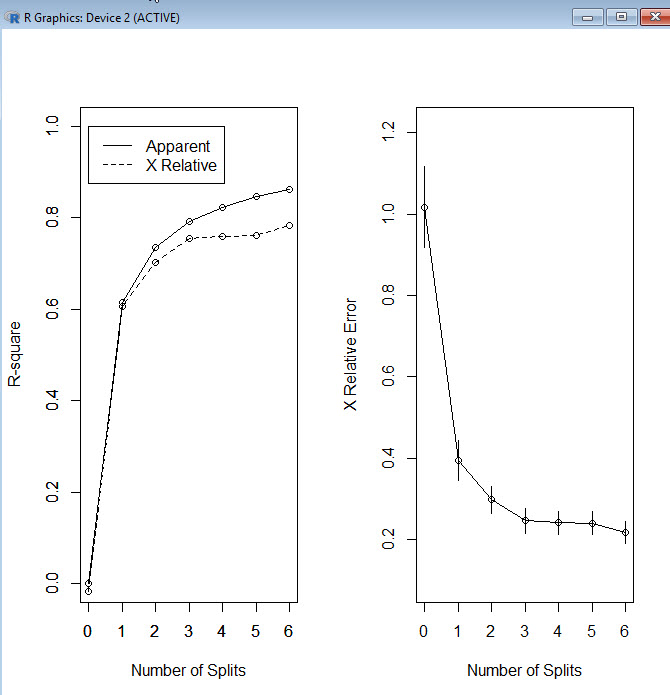Okay, so finally now that we know the model is good, let’s make a prediction.

```testData  <-data.frame (Species = 'setosa', Sepal.Width = 4, Petal.Length =1.2,
Petal.Width=0.3)
predict(fit, testData, method = "anova")```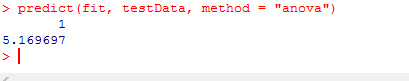So as you can see, based on our test data, the model predicts our Sepal.Length will be approx 5.17.

# R: Simple Linear Regression

Linear Regression is a very popular prediction method and most likely the first predictive algorithm most be people learn. To put it simply, in linear regression you try to place a line of best fit through a data set and then use that line to predict new data points.If you are new to linear regression or are in need of a refresher, check out my lesson on Linear Regression in Excel where I go much deeper into the mechanics: Linear Regression using Excel

## Get the Data

Let’s upload our file into R

```df <- read.csv(file.choose())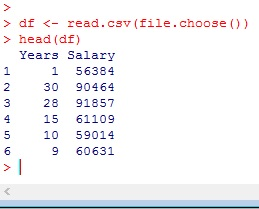Now our data file contains a listing of Years a person has worked for company A and their Salary.

### Check for linear relationship

With a 2 variable data set, often it is quickest just to graph the data to check for a possible linear relationship.

```#plot data
attach(df)
plot(Years, Salary)```

Looking at the plot, there definitely appears to be a linear relationship. I can easily see where I could draw a line through the data.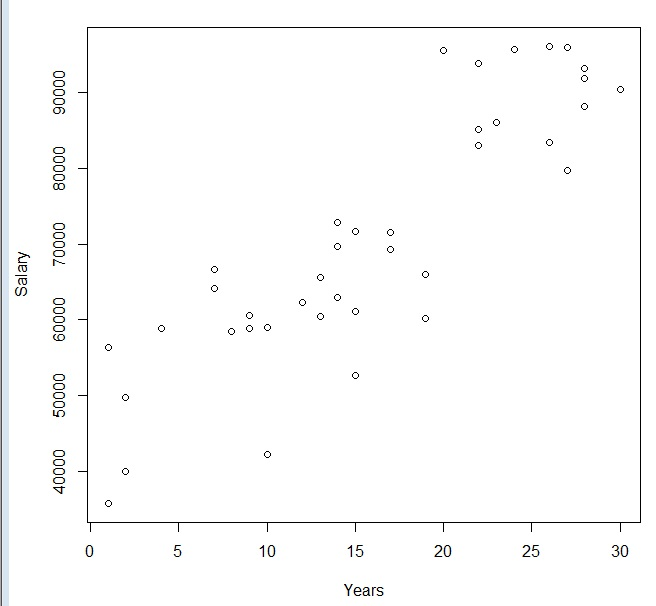An even better way to do it is to check for correlation. Remember the closer to 1, the better the correlation found in the data.

```#check for correlation
cor(Years, Salary)```Since our correlation is so high, I think it is a good idea to perform an linear regression.

## Linear Regression in R

A linear regression in R is pretty simple. The syntax is lm(y, x, data)

```#perform linear regression
fit <- lm(Salary~Years, data= df)
summary(fit)```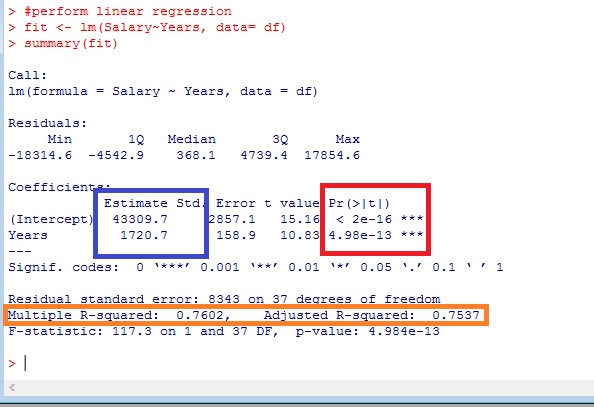Now let’s take a second to break down the output.

The red box shows my P values. I want to make sure they are under my threshold (usually 0.05). This becomes more important in multiple regression.

The orange box shows my R-squared values. Since this is a simple regression, both of these numbers are pretty much the same, and it really doesn’t matter which one you look at. What these numbers tell me is how accurate my prediction line is. A good way to look at them for a beginner is to consider them to be like percentages. So in our example, our prediction model is 75-76% percent accurate.

Finally, the blue box are your coefficients. You can use these numbers to create your predictive model. Remember the linear equation: Y = mX + b? Well using your coefficients here our equation now reads Y = 1720.7X + 43309.7

## Predictions

You can use fitted() to show you how your model would predict your existing data

`fitted(fit)`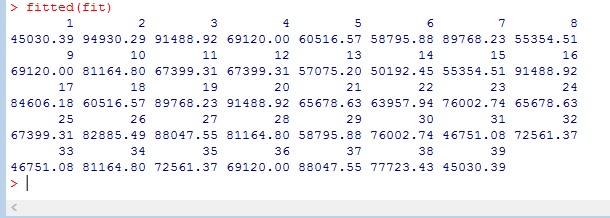You can also use the predict command to try a new value

`predict(fit, newdata =data.frame(Years= 40))`## Let’s graph our regression now.

```plot(Years, Salary)

abline(fit, col = 'red')```## The Residuals Plot

I am not going to go too deep into the weeds here, but I want to show you something cool.

```layout(matrix(c(1,2,3,4),2,2))  # c(1,2,3,4) gives us 4 graphs on the page,

#2,2 - graphs are 2x2
plot(fit)```

I promise to go more into this in a later lesson, but for now, I just want you to note the numbers you see popping up inside the graphs. (38,18,9) – These represent outliers. One of the biggest problems with any linear system is they are easily thrown off by outliers. So you need to know where you outliers are.If you look at the points listed in your graphs in your data, you will see why these are outliers. Now while this doesn’t tell you what to do about your outliers, that decision has to come from you, it is a great way of finding them quickly.## The Code

```# upload file

#plot data
attach(df)
plot(Years, Salary)

#check for correlation
cor(Years, Salary)

#perform linear regression
fit <- lm(Salary~Years, data= df)
summary(fit)

#see predictions
fitted(fit)

predict(fit, newdata =data.frame(Years= 40))

#plot regression line
plot(Years, Salary)

abline(fit, col = 'red')

layout(matrix(c(1,2,3,4),2,2))
plot(fit)

df```

# Logistic Regression with Gretl

One of the most popular machine learning algorithms, Logistic Regression is actually a classification algorithm. Broken down to its simplest terms, binary logistic regression (the one we will be focusing on here) is answering a yes or no question. Will the customer buy or not? Is the email SPAM or not?

## Score Accepted 982          0 1304         1 1256         1 1562         1 703          0

Above is a small sample from the data set we will be using for this lesson. In this set, student scores for an entrance test are listed in the first column and whether they were Accepted (1) or Not(0) is in the second column.

I ran a scatter plot on the data with Scores on the X axis. As you can see the dots for 2 horizontal lines at 1 and 0. You may notice that the 1 (Accepted) dots seem to cluster towards higher scores and 0 (Not Accepted) dots cluster towards lower scores.Well since the point of Logistic Regression is help us make predictions, here is how the predictions work. The Logistic Regression, represented by my crudely drawn red S, goes from 1 to 0. And just like with Linear Regression, if we take a value for X, to make our prediction, we look for the value of Y on the line at that point.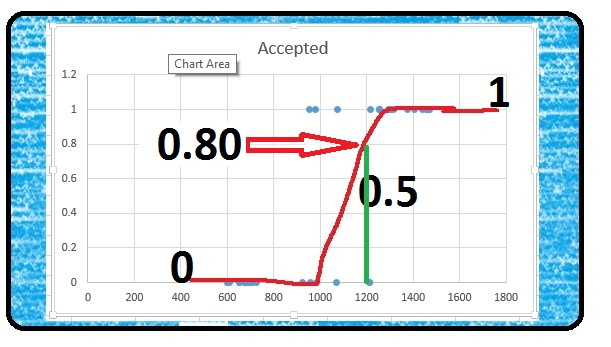In the case of a 1200 score, if we check the value of Y on the line, we get .80. This roughly translates to mean, that with a score of 1200, a student has an 80% chance of being accepted.

## Let’s meet Gretl

While there are third party add-ons you can download for Excel that will do Logistical Regression, in its native form, Excel does not do a good job in this area. So I thought this would be a great opportunity to introduce you to a neat piece of FREE software called Gretl.

So why Gretl? Why not R or Python? I mean those are the languages real data scientists use right?

That is true, and R and Python can easily do a Logical Regression. The problem is  however, in order to use R and Python, you need to know how to program. Gretl, on the other hand, is GUI based. Think of it as a point and click light weight R. It is no where near as robust as R, but for learning how to do Logistical Regression, Gretl does a fine job.

After you install and start Gretl, the next step is to load in the data. Go to File>Open Data>User File. Search for the Excel file you downloaded previously in this lesson. Make sure you then select Excel from the file type at the bottom of the screen.Select logi.xlsx. Leave the Start Import at window at 1 and 1. This is where the data starts in our Excel file: 1rst column, 1rst row. You will get a message letting you know how much data was imported.

The next pop up will noted that the data is undated. Click No on this window.You data columns (Score, Passed) will appear in the  Gretl window. If you click on one, the data from that column will appear in a pop-up window. **note in the file you download, column 2 will be Accepted not Passed.

## Let’s Model

Without further ado, let us do some modelings. From the menu bar Model>Limited dependent variable>Logit>Binary…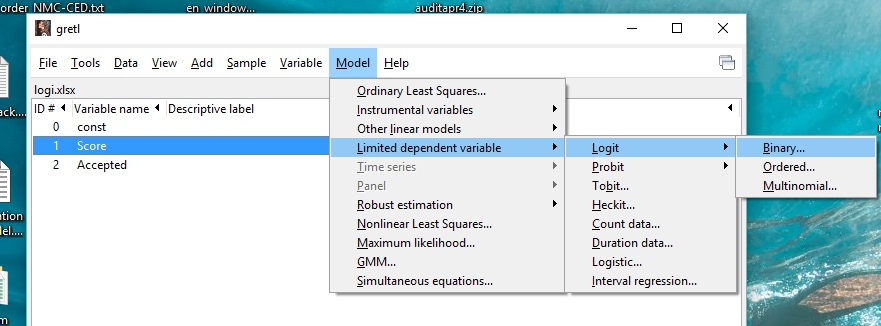Now you have to select you Dependent variable and Regressors. Here is a hint, the dependent variable is what we want to find. What are we looking for? Will the person be Accepted. So Accepted goes in Depentdent variable and Score goes in Regressors. Pick the Show p-values radio button and then click Okay.Below are the results of your Logistic Regression model

I am not going to give a Stats lesson here, but I will cover the important points.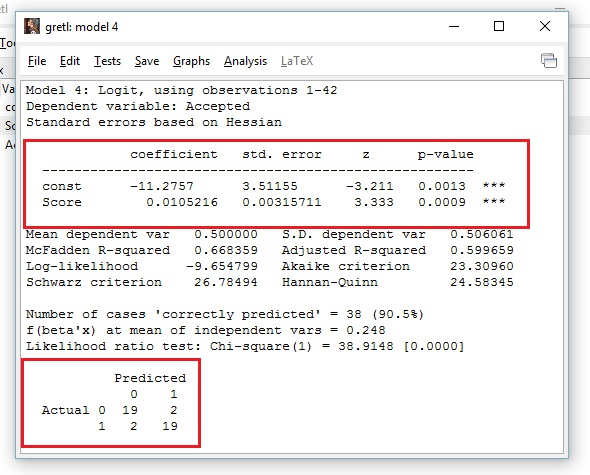1. The top red box contains some important information. First the coefficients represent the b and m values from the linear equation we will be using later: y=mX+b =y=0.0105216X + -11.2757
2. The p-value of Score = 0.0009 This is important as the p-value is a probabilistic value  that determines whether or not the regressor variable truly affects the dependent variable. The most common p-value threshold you are likely to come across is 0.05. If your regressor variable has a p-value above 0.05, you will want to reconsider your model.
3. The matrix at the bottom of the screen. This shows you how successfully your model predicted outcomes from the training data set. It translates of the 0’s (not accepted) the model got 19 out of 21 right. For 1’s(accepted) the model got 19 out of 21 right. That is a 90% success rate. Not bad.

## Let’s Use the Model

Okay, so maybe you jumped ahead and tried 1200 in the linear formula we developed above. It is 1.325?? How is that? Isn’t this supposed to be between 0 and 1.

Well the problem is, we are not looking for Y we are looking for probability (p). Y in this case is not the Y intercept, but instead: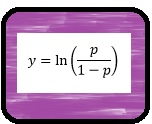Well, we know Y = 1.325 for a score of 1200, how do we find p from that? We solve  for p. Now feel free to go and do the math yourself if you want, but I already did the work for you. The equation below solves for p. If you don’t trust me and want to do it yourself, be my guest, but I assure you the equation below is good.## Let’s Make a Prediction

Let us put the formula’s we have found into ExcelNow you have a working prediction model. Any value you place in the score cell will be calculated to Y and p (probability). As the example above shows, a score of 1200 give us a probability of .79.

Turns out my crummy drawing wasn’t so bad after all.